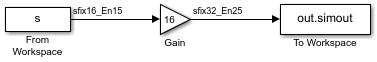## Pass Fixed-Point Data Between Simulink Models and MATLAB

You can read fixed-point data from the MATLAB® software into your Simulink® models, and there are several ways in which you can log fixed-point information from your models and simulations to the workspace.

### Read Fixed-Point Data from the Workspace

Use the From Workspace block to read fixed-point data from the MATLAB workspace into a Simulink model. To do this, the data must be in structure format with a Fixed-Point Designer™ `fi` object in the `values` field. In array format, the From Workspace block only accepts real, double-precision data.

To read in `fi` data, the Interpolate data parameter of the From Workspace block must not be selected, and the Form output after final data value by parameter must be set to anything other than `Extrapolation`.

### Write Fixed-Point Data to the Workspace

You can write fixed-point output from a model to the MATLAB workspace via the To Workspace block in either array or structure format. Fixed-point data written by a To Workspace block to the workspace in structure format can be read back into a Simulink model in structure format by a From Workspace block.

Note

To write fixed-point data to the workspace as a `fi` object, select the Log fixed-point data as a fi object check box on the To Workspace block dialog. Otherwise, fixed-point data is converted to `double` and written to the workspace as `double`.

For example, you can use the following code to create a structure in the MATLAB workspace with a `fi` object in the `values` field. You can then use the From Workspace block to bring the data into a Simulink model.

```a = fi([sin(0:10)' sin(10:-1:0)']) a = 0 -0.5440 0.8415 0.4121 0.9093 0.9893 0.1411 0.6570 -0.7568 -0.2794 -0.9589 -0.9589 -0.2794 -0.7568 0.6570 0.1411 0.9893 0.9093 0.4121 0.8415 -0.5440 0 DataTypeMode: Fixed-point: binary point scaling Signedness: Signed WordLength: 16 FractionLength: 15 s.signals.values = a s = struct with fields: signals: [1×1 struct] s.signals.dimensions = 2 s = struct with fields: signals: [1×1 struct] s.time = [0:10]' s = struct with fields: signals: [1×1 struct] time: [11×1 double]```

The From Workspace block in the following model has the `fi` structure `s` in the Data parameter. In the model, the following parameters in the Solver pane of the Configuration Parameters dialog box have the indicated settings:

• Start time`0.0`

• Stop time`10.0`

• Type`Fixed-step`

• Solver```discrete (no continuous states)```

• Fixed-step size (fundamental sample time)`1.0`The To Workspace block writes the result of the simulation to the MATLAB workspace as a `fi` structure.

```out.simout.data ans = 0 -8.7041 13.4634 6.5938 14.5488 15.8296 2.2578 10.5117 -12.1089 -4.4707 -15.3428 -15.3428 -4.4707 -12.1089 10.5117 2.2578 15.8296 14.5488 6.5938 13.4634 -8.7041 0 DataTypeMode: Fixed-point: binary point scaling Signedness: Signed WordLength: 32 FractionLength: 25```

### Log Fixed-Point Signals

When fixed-point signals are logged to the MATLAB workspace via signal logging, they are always logged as Fixed-Point Designer `fi` objects.

To enable signal logging, first select the signal. Then, in the Simulation tab, click .

When you log signals from a referenced model or Stateflow® chart in your model, the word lengths of `fi` objects may be larger than you expect. The word lengths of fixed-point signals in referenced models and Stateflow charts are logged as the next larger data storage container size.

### Access Fixed-Point Block Data During Simulation

Simulink provides an application programming interface (API) that enables programmatic access to block data, such as block inputs and outputs, parameters, states, and work vectors, while a simulation is running. You can use this interface to develop MATLAB programs capable of accessing block data while a simulation is running or to access the data from the MATLAB command line. Fixed-point signal information is returned to you via this API as `fi` objects. For more information about the API, refer to Access Block Data During Simulation.